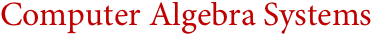# Computer Algebra Systems(Maple, Reduce, MuPAD, Magma, Axiom, Maxima, ...)
Computer algebra has been a central component of Mathematica's overall vision since its inception, and indeed Mathematica's original release in 1988 was what first brought computer algebra into the mainstream. For over 20 years, Wolfram Research has been a consistent leader in computer algebra research, implementing and inventing an unsurpassed number of new methods and algorithms—and indeed shaping the very concept of computer algebra.
While a variety of systems have been developed to provide basic computer algebra functionality, Mathematica is unique not only in delivering far greater depth and quality of coverage, but also in tightly integrating computer algebra into a unified overall framework. This integration is what has allowed Mathematica to bring computer algebra into industrial applications. And in education, it makes computer algebra an increasingly compelling educational tool, by linking it not only to the best in static visualization, but also now to a new generation of dynamic exploratory visualization and instant interactive interface creation technology. In addition, the Wolfram Demonstrations Project provides thousands of prebuilt resources for applying computer algebra in education.
In recent years, Mathematica's integration of efficient arbitrary-precision numerics, special functions, number theory, discrete mathematics, computational geometry and other areas has allowed Mathematica to drive the development of major new classes of computer algebra algorithms that could not realistically be implemented in narrowly defined computer algebra systems.
Computer Algebra System Features in Mathematica:
Key Advantages of Mathematica for Computer Algebra:
Interoperability with Computer Algebra Systems:
Interesting Tidbits:
• Wolfram Research has the world's most active computer algebra research organization
• Mathematica has been the basis for many thousands of mathematical papers
• Algorithms for Mathematica are increasingly discovered using NKS algorithm search methodology
• Mathematica has been a key element of calculus reform for nearly 20 years
• Mathematica was the first commercially successful system with computer algebra capabilities